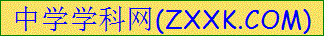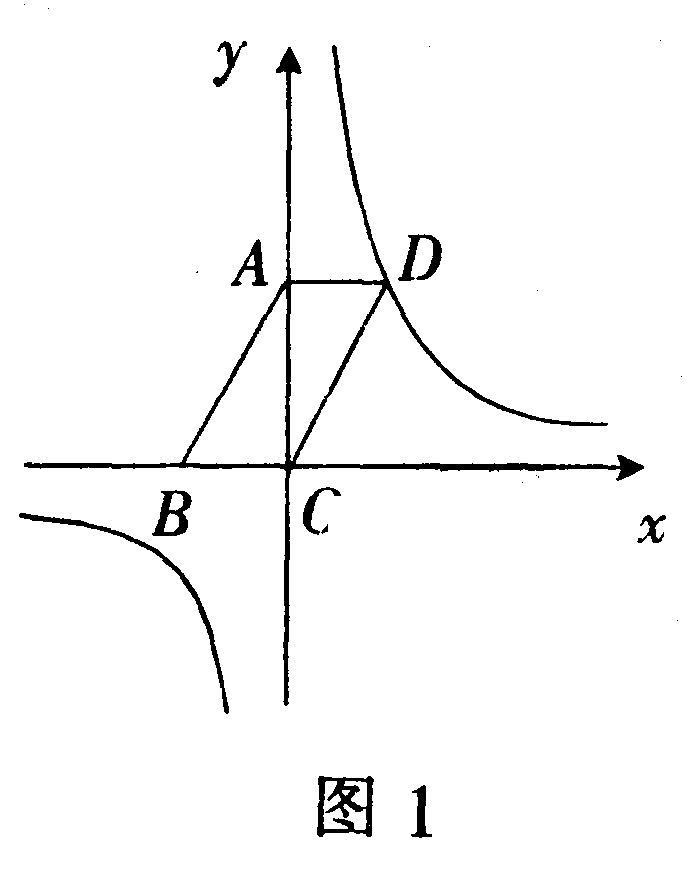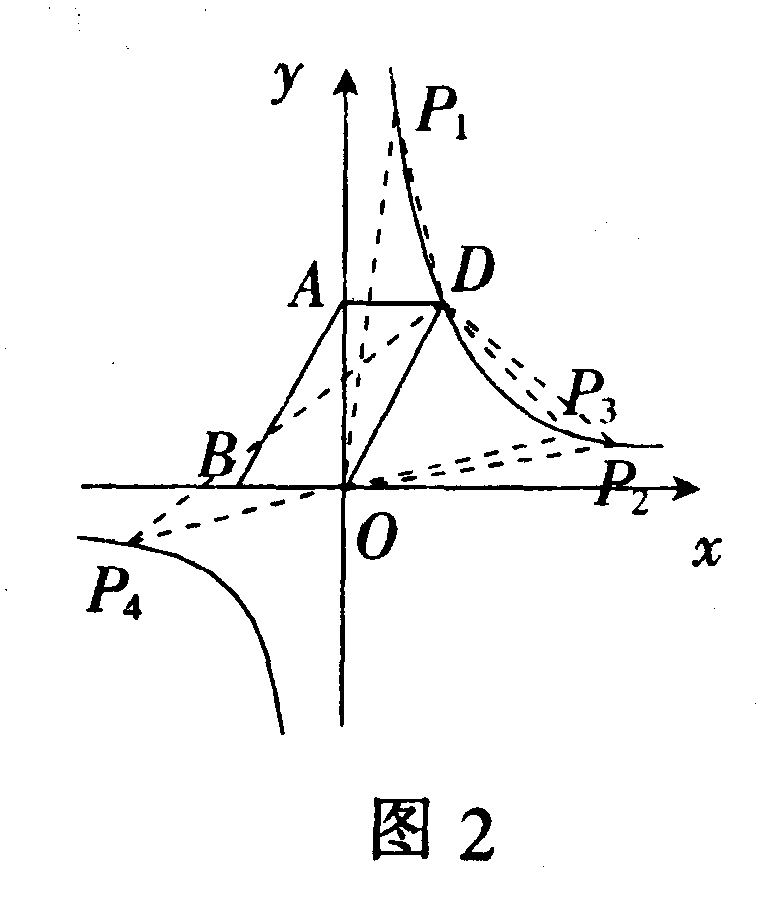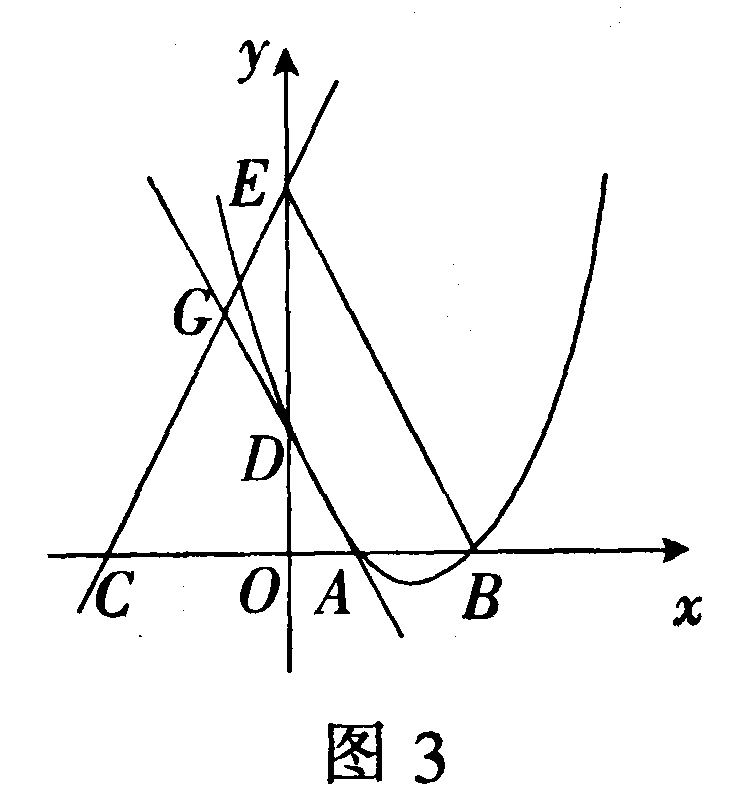# 中考数学教学指导：例谈参数在中考试题中的作用

纵观近年来各地中考试卷中，应用参数概念的题目常常出现，如在分式方程、一元二次方程、二次函数、反比例函数、方程组、不等式中等都有体现．同学们在复习时学会解决此类问题的方法十分必要．下面从近年各地中考题中精选几例供大家学习时参考．

一、含有参数的分式方程

(    )

(A) m>2

(B) m≥2

(C) m≥2且m≠3

(D) m>2且m≠3

简析  此题中，x是分式方程的未知数，m是未知数以外未知量，我们把m看作是此分式方程的参数．欲求出m的取值范围，可以运用解分式方程的方法，把分式方程去分母转化为整式方程，求出整式方程的解表示出x，根据已知条件方程的解为非负数，求出m的范围．此类题目在中考题中出现的频率较高，多为用参数确定分式方程解为正数为0，为非负数、为负数、有意义、无意义等．就此题来说，当解得x=m2之后，要注意(m2)1≠0和l(m2)≠0这个隐含条件．

分式方程去分母，得m3=xl，

解得x=m2．

由方程的解为非负数，得到

m2≥0，且m2≠1，

解得m>2且m≠3．

故选C

二、含有参数的一元二次方程

例2已知x1x2是关于x的一元二次方程x22(m + 1) x + m2+5=0的两实数根．

(1) 若(x11)( x21)=28，求m的值；

(2) 已知等腰△ABC的一边长为7，若x1x2恰好是△ABC另外两边的边长，求这个三角形的周长．

m2 + 52(m + 1)+1=28，解方程求得m的值；第 (2) 问分7为底边和7为腰两种情况分类讨论即可确定等腰三角形的周长．第 (1) 问解得m=4或m=6，要注意代回原方程检验，这一步非常重要，经检验当m=4时原方程无解；第 (2) 问分类讨论思想运用以及用求得的参数m的值检验三角形是否成立是解题难点，大家完成题目后要学会反思．[来源:学科网ZXXK]

(1) ∵ x1x2是关于x的一元二次方程x22(m + 1) x + m2 + 5=0的两实数根，

∴ x1+x2 =2(m + 1)，x1x2 =m2 + 5，

∴ (x11)( x21)

= x1x2(x1 + x2) + 1

= m2 + 52(m + 1) + l

= 28，

∴ m=6；

(2) ① 当7为底边时，此时方程x22(m + 1) x + m2 + 5=0有两个相等的实数根，

∴ △=4(m + 1)24(m2 + 5)=0，

解得m=2，

∴ 方程变为x26x + 9=0，

解得x1=x2=3．

∵ 3+3<7，∴ 不能构成三角形；

② 当7为腰时，设x1=7，代入方程，得4914(m + 1)+m2 + 5=0，

解得m=10，或4．

当m=10 时，方程变为

x222x+105=0，

解得x=7，或15．

∵ 7+7<15，故不能组成三角形．[来源:学科网ZXXK]

当m=4时方程变为x210x+21=0，

解得x=3或7，

此时三角形的周长为

7+7+3 =17．

三、含有参数的反比例函数

例3  已知反比例函数y=(m为常数) 的图像在一、三象限．

(1) 求m的取值范围；

(2) 如图1，若该反比例函数的图像经ABOD的顶点D，点A、B的坐标分别为 (0，3)，(2，0)．[来源:学科网]

① 求出函数解析式；

② 设点P是该反比例函数图像上的一点，若OD=OP，则P点的坐标为       ；若以D、O、P为顶点的三角形是等腰三角形，则满足条件的点P的个数为        个．

简析此题参数m在反比例函数“k”的位置，第 (1) 问可根据反比例函数的性质得出12m>0，然后解不等式得到m的取值范围；

② 根据反比例函数的图像关于原点中心对称可得点D关于原点的对称点P满足OP=OD，则此时P点坐标为 (2，3)；再根据反比例函数y=的图像关于直线y=x对称，可得点D (2，3) 关于直线y=x对称点P满足OP=OD．此时P点坐标为 (3，2)，易得点 (3，2) 关于原点的对称点P也满足OP=OD，此时P点坐标为 (3，2)．由于以D、O、P为顶点的三角形是等腰三角形，所以以D点为顶点可画出点P1P2，以O点顶点可画出点P3P4，如图2．此题涉及的知识点有反比例函数图像的性质和其图像上点的坐标特征、平行四边形的性质和等腰三角形的性质等．(略)．

四、含有参数的二次函数[来源:学&科&网Z&X&X&K][来源:学§科§网]

例4已知抛物线y=x2(k + 2) x + 和直线y=(k + 1) x + (k + 1)2

(1) 求证：无论k取何实数值，抛物线总与x轴有两个不同的交点；

(2) 抛物线与x轴交于点A、B，直线与x轴交于点C，设A、B、C三点的横坐标分别是x1x2x3，求x1x2x3的最大值；

(3) 如果抛物线与x轴的交点A、B在原点的右边，直线与x轴的交点C在原点的左边，又抛物线、直线分别交y轴于点D、E，直线AD交直线CE于点G (如图3)，且CA×GE=CG×AB，求抛物线的解析式．简析  第(1) 问由一元二次方程根的判别式大于0时，方程有两个不相等的实数根，有△= (k + 2)2－4×1×

= k2k + 2

= (k)2+>0．

通过证明证得无论k取何实数值，抛物线总与x轴有两个不同的交点．

第(2) 问由抛物线于x轴交于点A、B，直线与x轴交于点C，设A、B、C三点的横坐标分别是x1x2x3，可得x1x2=．由已知直线y=(k + 1) x + (k + 1)2x轴交于点C，令y=0，x3=(k + 1)，进而可求得答案．

OA·OB=

OD=OE=(k + 1)2

进而求得点B的坐标为 (k + 1，0)，代入解析式即可求得答案．

此题主要考查了一次函数与二次函数的性质、待定系数法求函数的解析式以及相似三角形的判定与性质．数学思想是数形结合思想与方程思想的应用．

五、含有参数重新规定运算符号的问题

例5 xy定义一种新运算T，规定：T (xy)=(其中ab均为非零常数)，这里等式右边是通常的四则运算，例如：T (0，1) == b

(1) 已知T (1，1)=2，T (4，2)=1．

① 求ab的值；

② 若关于 m 的不等式组恰好有3个整数解，求实数p的取值范围；

(2) 若T (xy)=T (yx) 对任意实数xy都成立 (这里T (xy) 和T (yx) 均有意义)，则ab应满足怎样的关系式?

简析  第(1) 问根据给出新的运算规定，① 已知两对值代入T中，得出两个含有ab的二元一次方程，组成方程组即可计算求出ab的值；② 继续运用新的运算规定，把相应的代数式代入、化简得到新的不等式，根据不等式组恰好有3个整数解，求出p的范围即可；

第(2) 问把xy代入到新运算中，由T (xy)=T (yx)列出关系式，整理后得到

(x2y2)(2ba)=0，

由已知条件T (xy)=T (yx) 对任意实数xy都成立，即可确定出ab的关系式．此题对xy定义一种新运算T，作出新的运算规定，根据新运算规定，由浅入深，步步为营，不但使解题思维连贯，而且逻辑性非常强，是此类题目的典型代表之一．